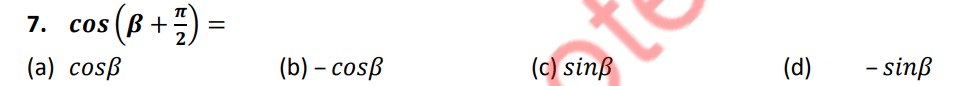# FSC Math Full Book MCQs 4

NUST entry test 2023 NET contains most of the Mathematics MCQs. FSC Part 2 Math book MCQs. HSSC Math questions MCQs. Solved Chapterwise Math MCQs for first-year 12 class MCQs. Second-year and first-year Math textbooks chapter-wise MCQs with the answer are provided here. PakLearningSpot PLS MCQs Bank website for FREE Entry test preparations

0%
74
Created by Ali Durrani

NET Practice

Math Practice Test 4

1 / 100

Category: Math Ch 3

Which of the following Sets is not a field.

2 / 100

Category: Math Ch 7

Let 𝑺 = {𝟏, 𝟐, 𝟑, … , 𝟏𝟎} the probability that a number is divided by 4 is :

3 / 100

Category: Math Ch 4

The sum of all four fourth roots of unity is:

4 / 100

Category: Math Ch 12

The circle passing through the thee vertices of a triangle is called:

5 / 100

Category: Math Ch 1

(−𝟏)^−𝟐𝟏/𝟐 =

6 / 100

Category: Logical Reasoning – Logical problems

The high school math department needs to appoint a new chairperson, which will be based on seniority. Ms. West has less seniority than Mr. Temple, but more than Ms. Brody. Mr. Rhodes has more seniority than Ms. West, but less than Mr. Temple. Mr. Temple doesn’t want the job. Who will be the new math department chairperson?

PreMedPK MDCAT Mock 5
Logical Reasoning
Logical Problems
Passage
Elimination Tool:

7 / 100

Category: Math Ch 7 Class 12

The lines joining the mid-points of any two sides of a triangle is always _____to the third side.

8 / 100

Category: Math Ch 12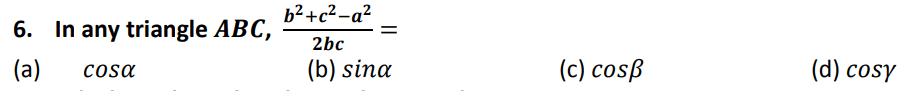9 / 100

Category: Math Ch 10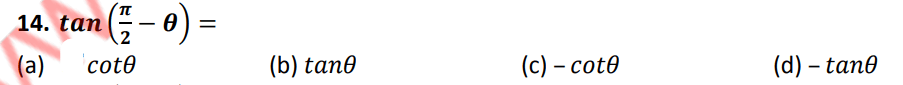10 / 100

Category: Math Ch 14

All trigonometric functions are ……………….. functions.

11 / 100

Category: Math Ch 5 Class 12

(0,0) is the solution of the inequality

12 / 100

Category: Math Ch 7

If an event always occurs , then it is called:

13 / 100

Category: Math Ch 3 Class 12

The equation 𝒚 = 𝒙𝟐 − 𝟐𝒙 + 𝒄 represents ( 𝒄 being a parameter )
(Error Comment below the anwser)

14 / 100

Category: Math Ch 3

10. If a matrix A has different number of rows and columns then A is called:

15 / 100

Category: Math Ch 5 Class 12

The solution of 𝒂𝒙 + 𝒃 < 𝑐 is

16 / 100

Category: Math Ch 6

. An infinite geometric series is divergent if

17 / 100

Category: Math Ch 2

𝒑 → 𝒒 is called converse of

18 / 100

Category: Math Ch 319 / 100

Category: Math Ch 6 Class 12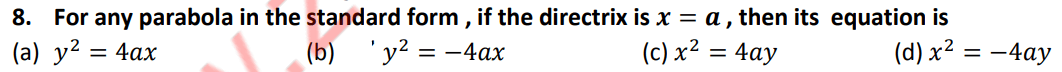20 / 100

Category: Math Ch 4 Class 12

𝑰𝒇 𝒙 < 0, 𝑦 < 0 then the point 𝑷(𝒙, 𝒚) lies in the quadrant

21 / 100

Category: Math Ch 1422 / 100

Category: Math Ch 7 Class 12

Parallelogram law of vector addition to describe the combined action of two forces, was used
by

23 / 100

Category: Math Ch 3

A rectangular array of numbers enclosed by a square brackets is called:

24 / 100

Category: Math Ch 425 / 100

Category: Math Ch 3

If a matrix A has only one column then it is called:

26 / 100

Category: Math Ch 4

9. Sum of all cube roots of 64 is :

27 / 100

Category: Math Ch 3

The horizontal lines of numbers in a matrix are called:

28 / 100

Category: Math Ch 3 Class 12

∫?′(?)/?(?) ?? =

29 / 100

Category: Math Ch 630 / 100

Category: Math Ch 4

11. 𝟏𝟔𝝎^𝟖 + 𝟏𝟔𝒘^𝟒 =

31 / 100

Category: Math Ch 11

Period of 𝒕𝒂𝒏𝟒𝒙 is

32 / 100

Category: Math Ch 2 Class 12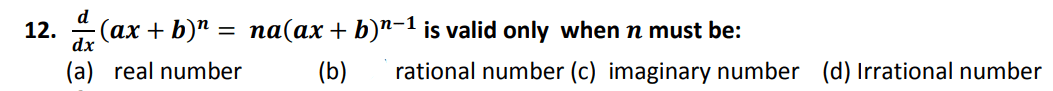33 / 100

Category: Math Ch 6

No term of a 𝑮. 𝑷., is:

34 / 100

Category: Math Ch 7 Class 1235 / 100

Category: Math Ch 1 Class 12

When we say that 𝒇 is function from set 𝑿 to set 𝒀, then 𝑿 is called

36 / 100

Category: Math Ch 4

Product of cube roots of -1 is:

37 / 100

Category: Math Ch 3 Class 12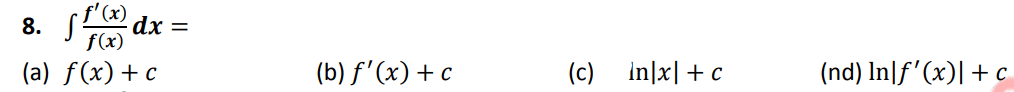38 / 100

Category: Math Ch 5 Class 12

Corner point is also called

39 / 100

Category: Math Ch 7 Class 12

The angles 𝜶, 𝜷, 𝒂𝒏𝒅 𝜸 which a non-zero vector 𝒓 makes with 𝒙 − 𝒂𝒙𝒊𝒔, 𝒚 − 𝒂𝒙𝒊𝒔 and
𝒛 − 𝒂𝒙𝒊𝒔 respectively are called_____________ of 𝒓.

40 / 100

Category: Math Ch 6

A sequence is also known as:

41 / 100

Category: Math Ch 4 Class 12

The point where the altitudes of a triangle intersect is called_________ of the triangle.

42 / 100

Category: Math Ch 10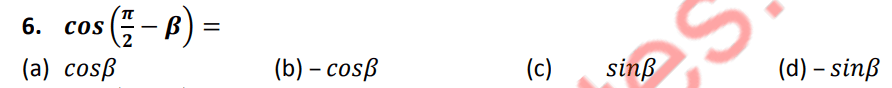43 / 100

Category: Math Ch 3

4. If a matrix A has 𝒎 rows and 𝒏 columns , then order of A is :

44 / 100

Category: Math Ch 6 Class 12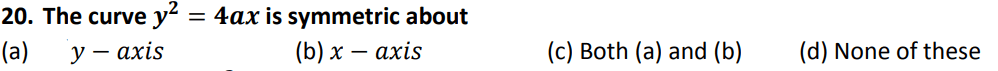45 / 100

Category: Math Ch 3 Class 1246 / 100

Category: Math Ch 2 Class 12

A stationary point is called ______ if it is either a maximum point or a minimum point

47 / 100

Category: Math Ch 2 Class 12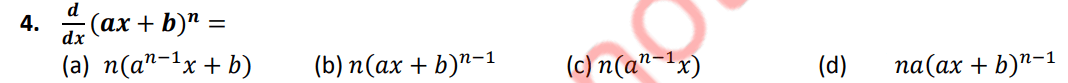48 / 100

Category: Math Ch 8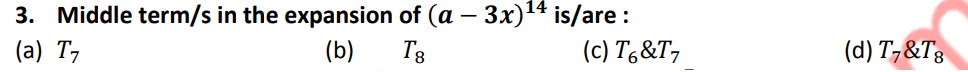49 / 100

Category: Math Ch 11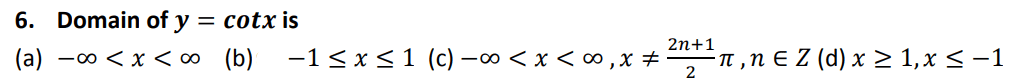50 / 100

Category: Math Ch 1 Class 12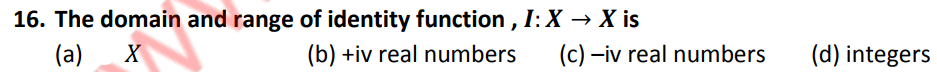51 / 100

Category: Math Ch 14

General solution of every trigonometric equation consists of :

52 / 100

Category: Math Ch 7 Class 12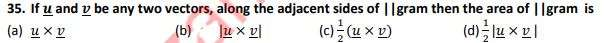53 / 100

Category: Math Ch 4

The sum of all four fourth roots of 16 is:

54 / 100

Category: Math Ch 5 Class 12

(0,0) is satisfied by

55 / 100

Category: Math Ch 1

For any complex number 𝒛, it is always true that |𝒛| is equal to:

56 / 100

Category: Math Ch 4 Class 12

The straight line which passes through one vertex and perpendicular to opposite side is
called:

57 / 100

Category: Math Ch 1258 / 100

Category: Math Ch 3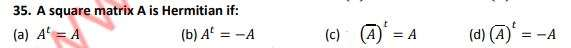59 / 100

Category: Math Ch 4

Basic techniques for solving quadratic equations are

60 / 100

Category: Math Ch 9

Two rays with a common starting point form:

61 / 100

Category: Math Ch 4 Class 12

The Normal form of the equation of the straight line is

62 / 100

Category: Math Ch 8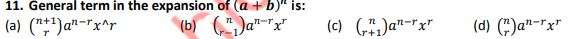63 / 100

Category: Math Ch 7 Class 12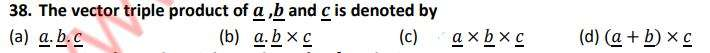64 / 100

Category: Math Ch 3

If a matrix of order ? × ? then the matrix of order ? × ? is :

65 / 100

Category: Math Ch 1 Class 12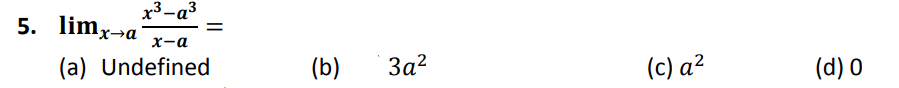66 / 100

Category: Math Ch 7 Class 12

Two vectors are said to be negative of each other if they have the same magnitude and
__________direction.

67 / 100

Category: Math Ch 5

Which is a reducible factor:

68 / 100

Category: Math Ch 13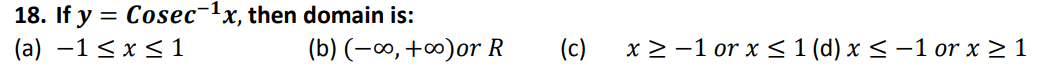69 / 100

Category: Math Ch 7 Class 1270 / 100

Category: Math Ch 4

The complex fourth roots of unity are ……. of each other.

71 / 100

Category: Math

A compound proposition which is always neither true nor false is called:

72 / 100

Category: Math Ch 5

An open sentence formed by using sign of “ = ” is called a/an

73 / 100

Category: Math Ch 5 Class 12

(0,0) is the solution of the inequality

74 / 100

Category: Math Ch 5 Class 12

The graph of corresponding linear equation of the linear inequality is a line called________

75 / 100

Category: Math Ch 2

The set of odd numbers between 1 and 9 are

76 / 100

Category: Math Ch 7 Class 12

The angle in semi-circle is equal to:

77 / 100

Category: Math Ch 14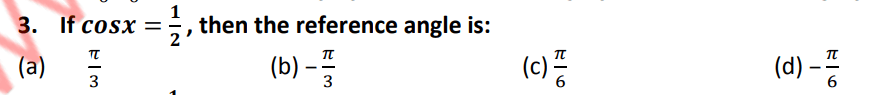78 / 100

Category: Math Ch 379 / 100

Category: Math Ch 1 Class 12

The term “Function” was recognized by______ to describe the dependence of one quantity
to another.

80 / 100

Category: Math Ch 6 Class 12

The set of points which are equal distance from a fixed point is called:

81 / 100

Category: Math Ch 2 Class 1282 / 100

Category: Math Ch 7 Class 12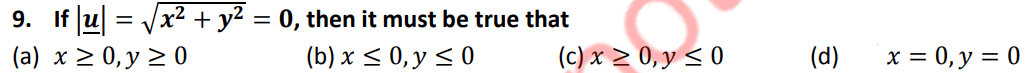83 / 100

Category: Math

Every function is:

84 / 100

Category: Math Ch 7 Class 12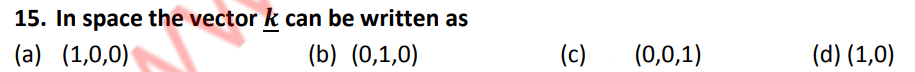85 / 100

Category: Math Ch 1386 / 100

Category: Math Ch 7 Class 12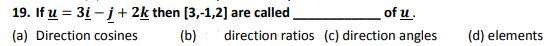87 / 100

Category: Math Ch 3 Class 12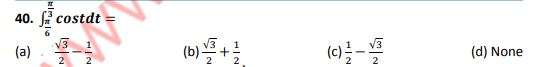88 / 100

Category: Math Ch 10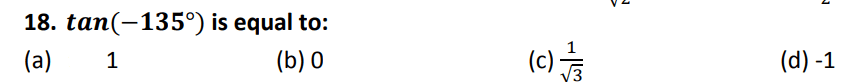89 / 100

Category: Math Ch 7 Class 12

. If the scalar product of three vectors is zero, then vectors are

90 / 100

Category: Math Ch 5 Class 12

A function which is to be maximized or minimized is called______ function

91 / 100

Category: Math Ch 3 Class 12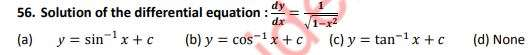92 / 100

Category: Math

. Truth set of a tautology is

93 / 100

Category: Math Ch 7

Sample space for tossing a coin is:

94 / 100

Category: Math Ch 795 / 100

Category: Math Ch 1

The multiplicative inverse of (𝟒, −𝟕) is:

96 / 100

Category: Math Ch 13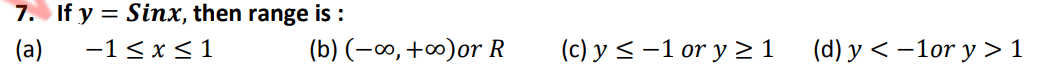97 / 100

Category: Math Ch 2 Class 12

A point where 1st derivative of function is zero , is called

98 / 100

Category: Math Ch 1199 / 100

Category: Math Ch 12

7. Which can be reduced to Pythagoras theorem,

100 / 100

Category: Math Ch 10